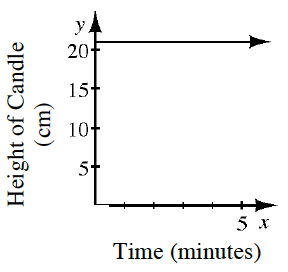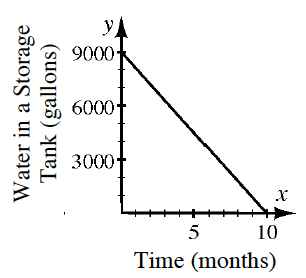### Home > CC4 > Chapter 2 > Lesson 2.2.2 > Problem2-63

2-63.

Explain what the slope of each line below represents. Then determine the slope and give its units. Homework Help ✎

1.The slope represents the change in height of a candle per minute.

The line is horizontal and shows no change; therefore, the slope is zero and the candle is not burning.

1.The slope represents the gallons per month of water being removed from a well.

Using a slope triangle, find the distances of both legs of the triangle. Remember that the slope is the rise over the run, or the change in $y$ over the change in $x$.